# 激活函数¶

## 什么是激活函数¶

• 激活函数是人工神经网络的一个极其重要的特征；

• 激活函数决定一个神经元是否应该被激活，激活代表神经元接收的信息与给定的信息有关；

• 激活函数对输入信息进行非线性变换，然后将变换后的输出信息作为输入信息传给下一层神经元。

## 激活函数的种类¶

### identity¶

$f(x)=x$

${ f }^{ ' }(x)=1$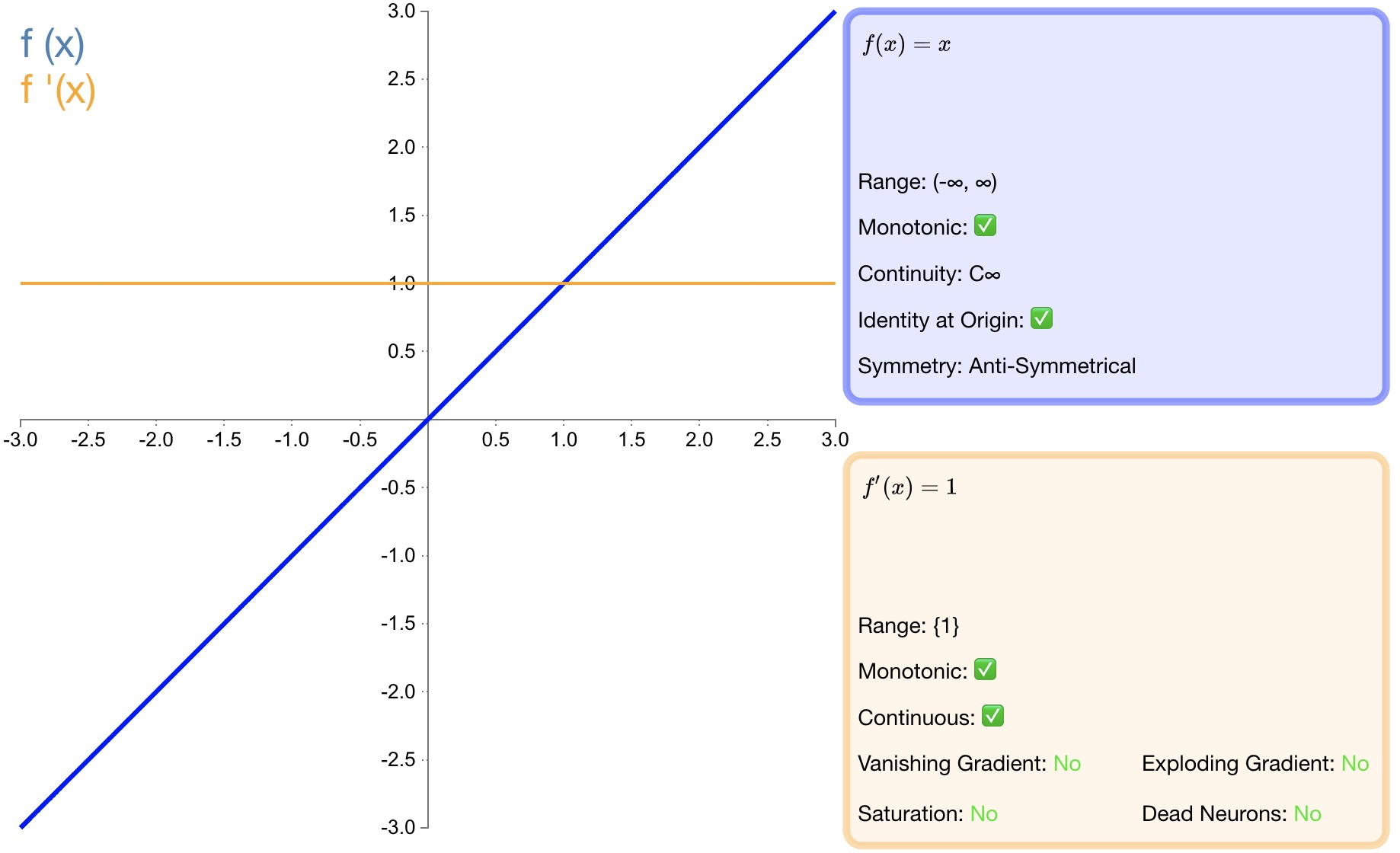### step¶

$\begin{split} { f }(x)=\begin{cases} \begin{matrix} 0 & x<0 \end{matrix} \\ \begin{matrix} 1 & x\ge 0 \end{matrix} \end{cases}\end{split}$

$\begin{split} { f }^{ ' }(x)=\begin{cases} \begin{matrix} 0 & x\neq 0 \end{matrix} \\ \begin{matrix} ? & x=0 \end{matrix} \end{cases}\end{split}$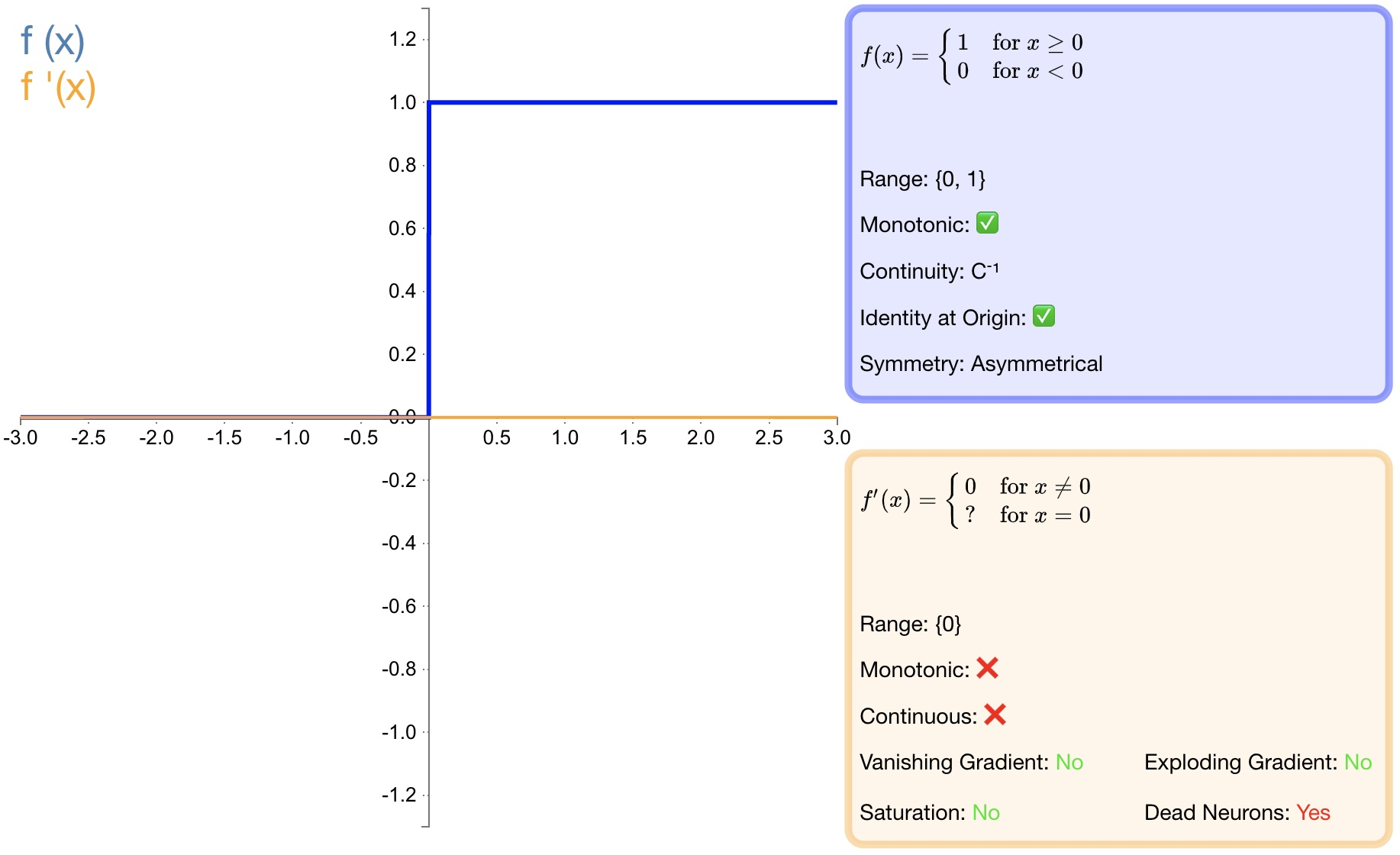### sigmoid¶

${ f }(x)=\sigma (x)=\frac { 1 }{ 1+{ e }^{ -x } }$

${ f }^{ ' }(x)=f(x)(1-f(x))$1. $$sigmoid$$ 函数的输出映射在 $$(0,1)$$ 之间，单调连续，输出范围有限，优化稳定，可以用作输出层；

2. 求导容易；

1. 由于其软饱和性，一旦落入饱和区梯度就会接近于0，根据反向传播的链式法则，容易产生梯度消失，导致训练出现问题；

2. Sigmoid函数的输出恒大于0。非零中心化的输出会使得其后一层的神经元的输入发生偏置偏移（Bias Shift），并进一步使得梯度下降的收敛速度变慢；

3. 计算时，由于具有幂运算，计算复杂度较高，运算速度较慢。

### tanh¶

${ f }(x)=tanh(x)=\frac { { e }^{ x }-{ e }^{ -x } }{ { e }^{ x }+{ e }^{ -x } }$

${ f }^{ ' }(x)=1-f(x)^{ 2 }$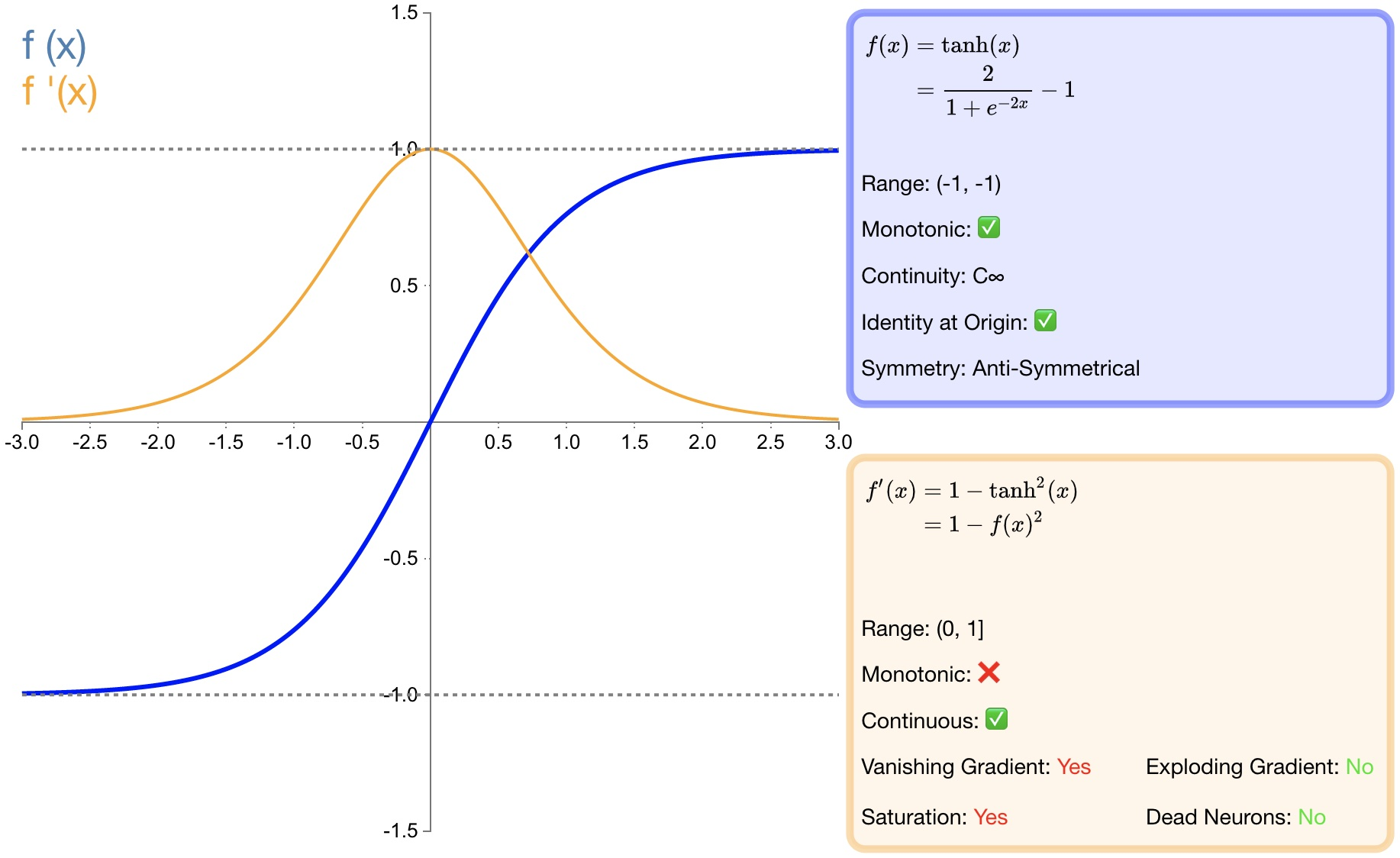1. $$tanh$$$$sigmoid$$ 函数收敛速度更快；

2. 相比 $$sigmoid$$ 函数，$$tanh$$ 是以 $$0$$ 为中心的；

1. $$sigmoid$$ 函数相同，由于饱和性容易产生的梯度消失；

2. $$sigmoid$$ 函数相同，由于具有幂运算，计算复杂度较高，运算速度较慢。

### ReLU¶

$\begin{split}f(x)=\begin{cases} \begin{matrix} 0 & x<0 \end{matrix} \\ \begin{matrix} x & x\ge 0 \end{matrix} \end{cases}\end{split}$

$\begin{split}{ { f }(x) }^{ ' }=\begin{cases} \begin{matrix} 0 & x<0 \end{matrix} \\ \begin{matrix} 1 & x\ge 0 \end{matrix} \end{cases}\end{split}$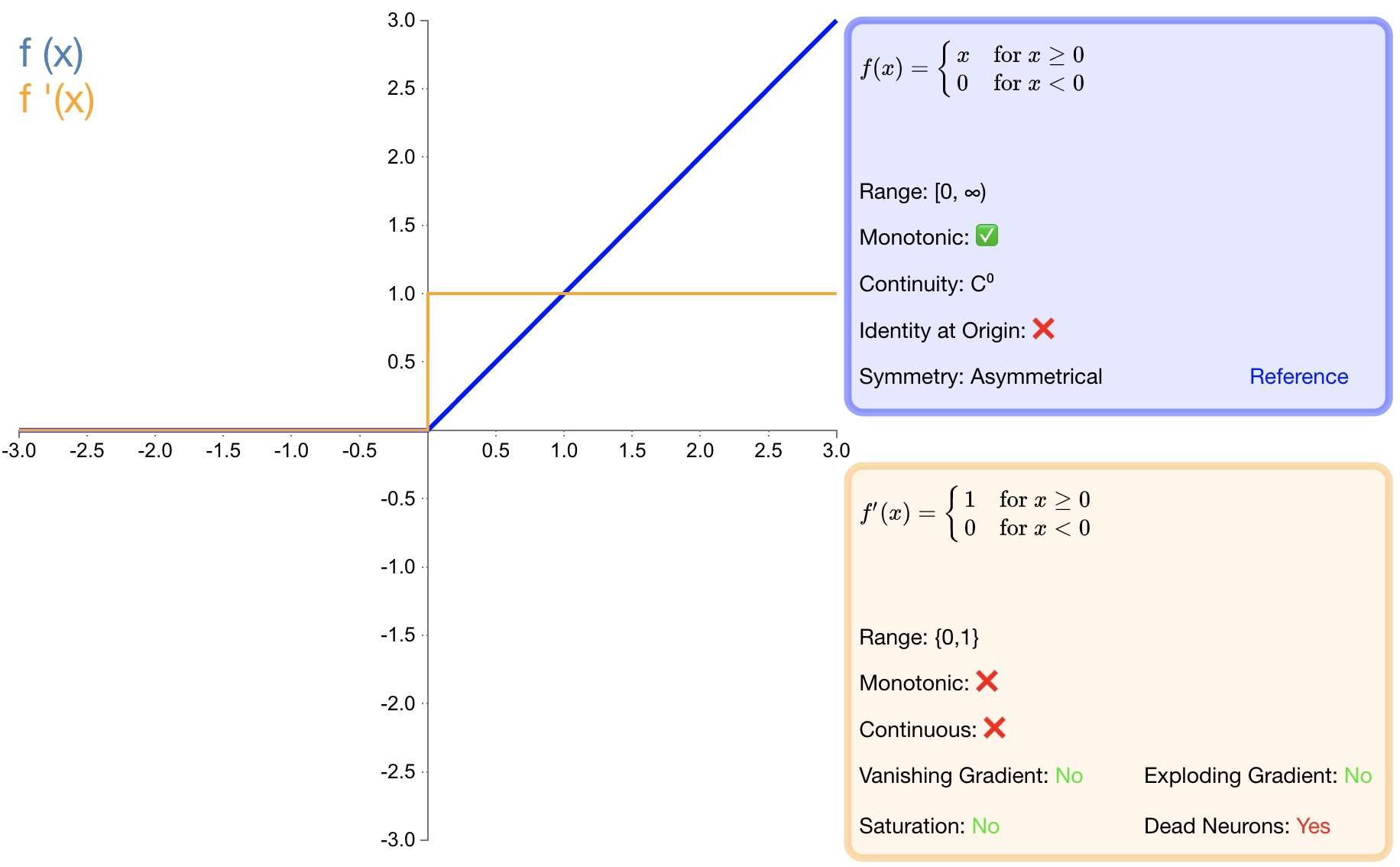1. 收敛速度快；

2. 相较于 $$sigmoid$$$$tanh$$ 中涉及了幂运算，导致计算复杂度高， ReLU​可以更加简单的实现；

3. 当输入 $$x>=0$$ 时，ReLU​ 的导数为常数，这样可有效缓解梯度消失问题；

4. $$x<0$$ 时，ReLU​ 的梯度总是 $$0$$，提供了神经网络的稀疏表达能力；

1. ReLU​ 的输出不是以 $$0$$ 为中心的；

2. 神经元坏死现象，某些神经元可能永远不会被激活，导致相应参数永远不会被更新；

3. 不能避免梯度爆炸问题；

### LReLU¶

$\begin{split} f(x)=\begin{cases} \begin{matrix} \alpha x & x<0 \end{matrix} \\ \begin{matrix} x & x\ge 0 \end{matrix} \end{cases}\end{split}$

$\begin{split}{ { f }(x) }^{ ' }=\begin{cases} \begin{matrix} \alpha & x<0 \end{matrix} \\ \begin{matrix} 1 & x\ge 0 \end{matrix} \end{cases}\end{split}$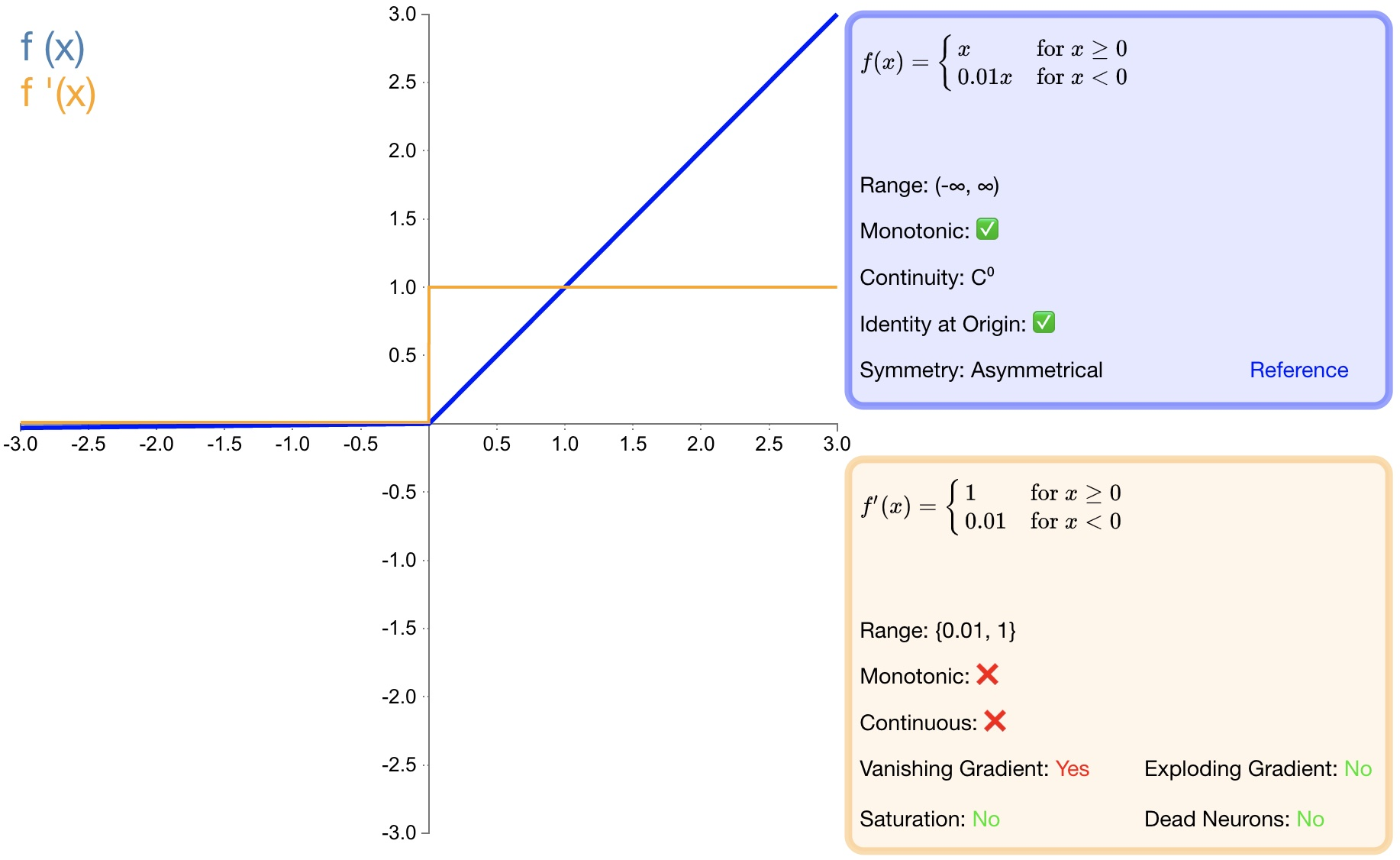1. 避免梯度消失；

2. 由于导数总是不为零，因此可减少死神经元的出现；

1. LReLU​ 表现并不一定比 ReLU​ 好；

2. 无法避免梯度爆炸问题；

### PReLU¶

$\begin{split}f(\alpha ,x)=\begin{cases} \begin{matrix} \alpha x & x<0 \end{matrix} \\ \begin{matrix} x & x\ge 0 \end{matrix} \end{cases}\end{split}$

$\begin{split}{ { f }(\alpha ,x) }^{ ' }=\begin{cases} \begin{matrix} \alpha & x<0 \end{matrix} \\ \begin{matrix} 1 & x\ge 0 \end{matrix} \end{cases}\end{split}$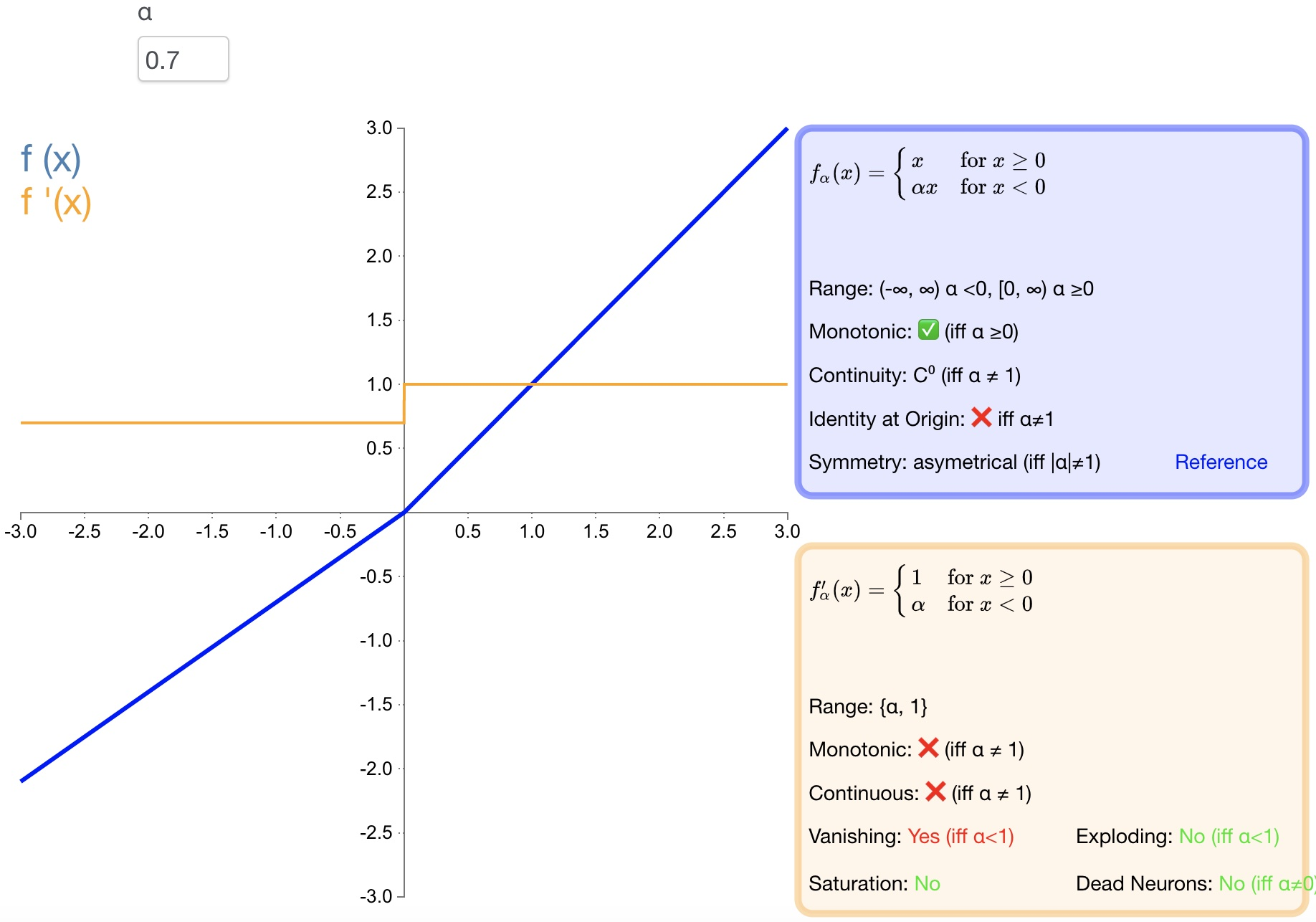1. PReLU​ 是 LReLU 的改进，可以自适应地从数据中学习参数；

2. 收敛速度快、错误率低；

3. PReLU 可以用于反向传播的训练，可以与其他层同时优化；

### RReLU¶

$\begin{split}f(\alpha ,x)=\begin{cases} \begin{matrix} \alpha & x<0 \end{matrix} \\ \begin{matrix} x & x\ge 0 \end{matrix} \end{cases}\end{split}$

$\begin{split}{ { f }(\alpha ,x) }^{ ' }=\begin{cases} \begin{matrix} \alpha & x<0 \end{matrix} \\ \begin{matrix} 1 & x\ge 0 \end{matrix} \end{cases}\end{split}$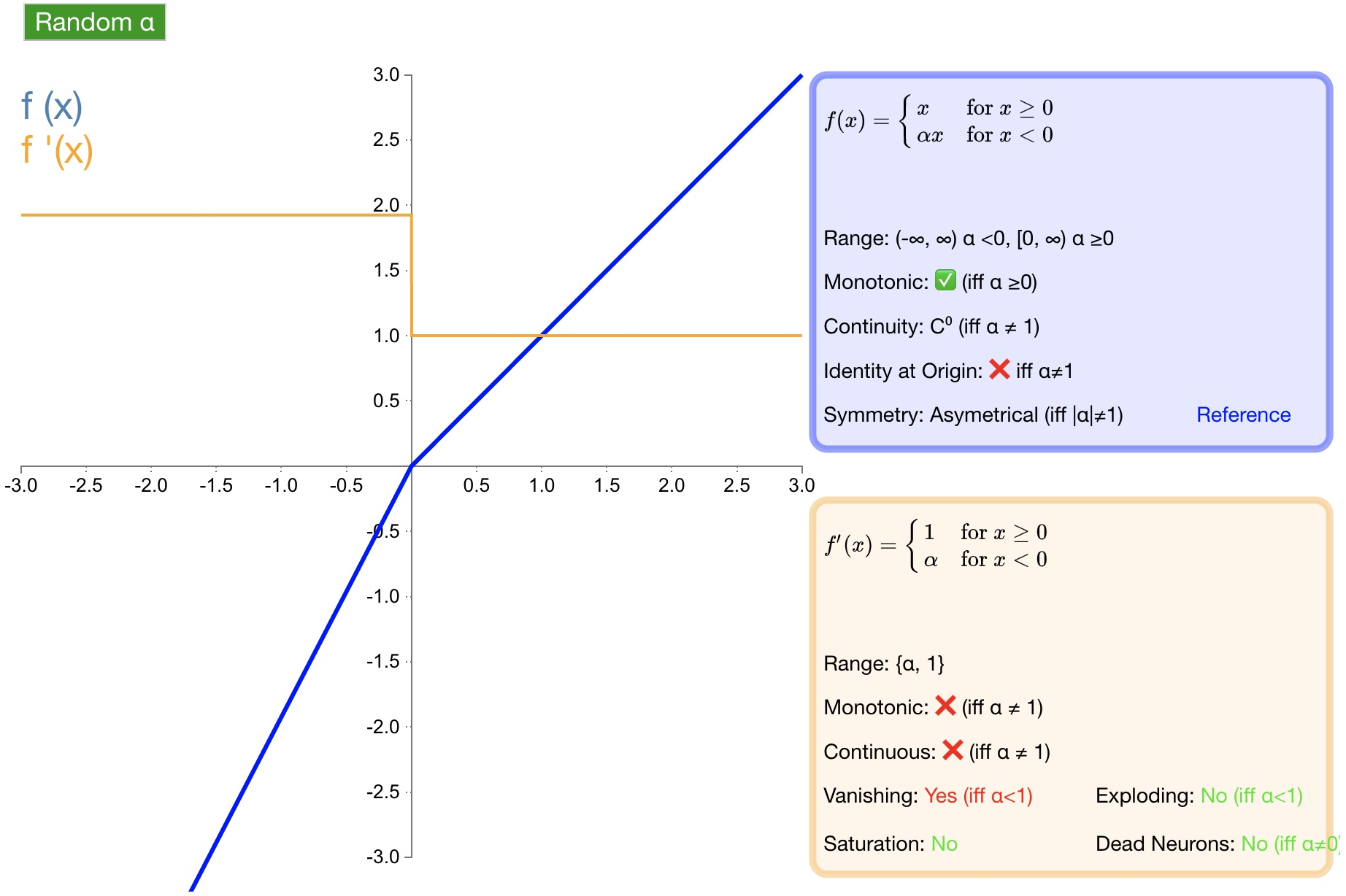### ELU¶

$\begin{split} f(\alpha ,x)=\begin{cases} \begin{matrix} \alpha \left( { e }^{ x }-1 \right) & x<0 \end{matrix} \\ \begin{matrix} x & x\ge 0 \end{matrix} \end{cases}\end{split}$

$\begin{split}{ { f }(\alpha ,x) }^{ ' }=\begin{cases} \begin{matrix} f(\alpha ,x)+\alpha & x<0 \end{matrix} \\ \begin{matrix} 1 & x\ge 0 \end{matrix} \end{cases}\end{split}$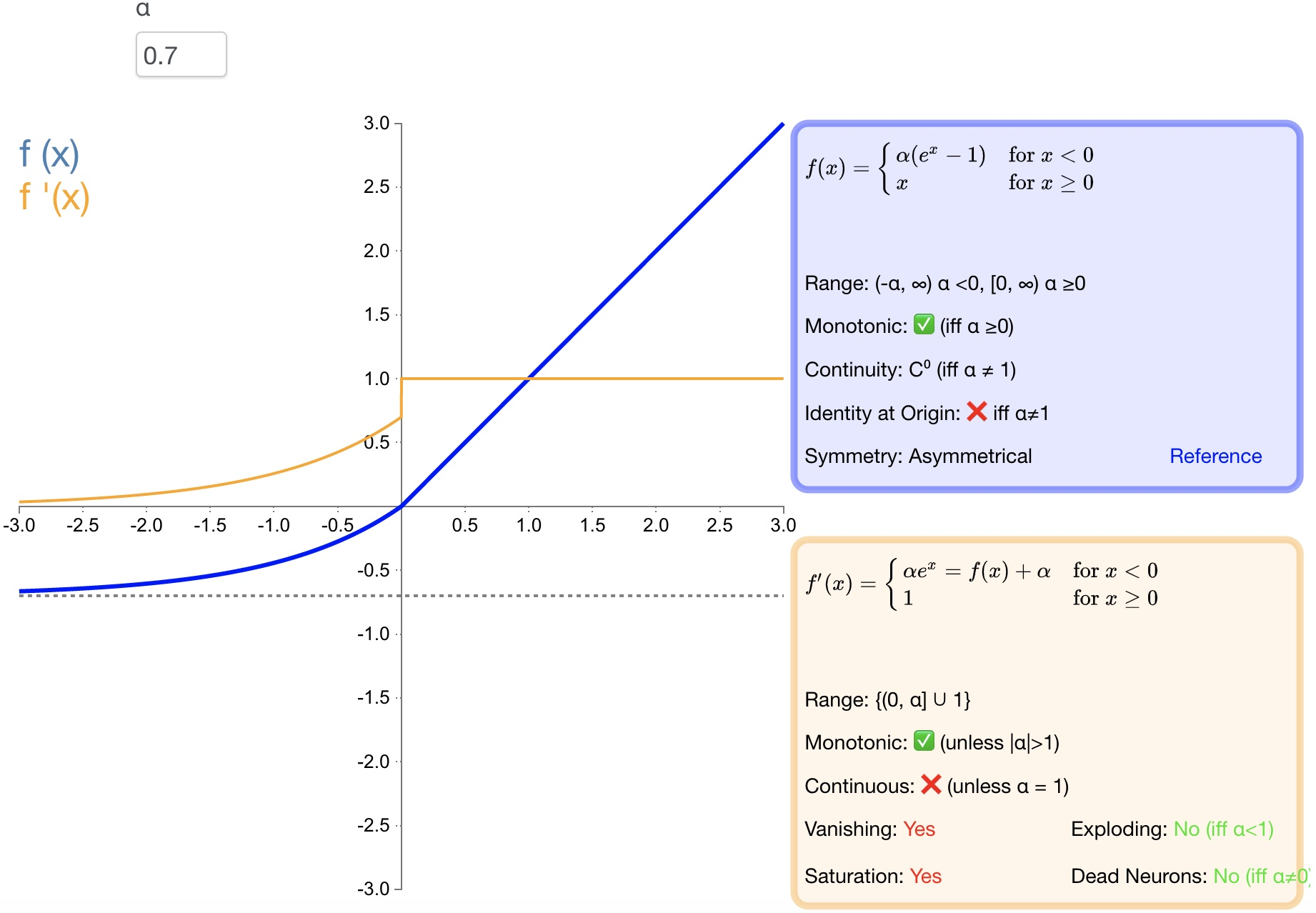1. 导数收敛为零，从而提高学习效率；

2. 能得到负值输出，这能帮助网络向正确的方向推动权重和偏置变化；

3. 防止死神经元出现。

1. 计算量大，其表现并不一定比 ReLU 好；

2. 无法避免梯度爆炸问题；

### SELU¶

$\begin{split}f(\alpha ,x)=\lambda \begin{cases} \begin{matrix} \alpha \left( { e }^{ x }-1 \right) & x<0 \end{matrix} \\ \begin{matrix} x & x\ge 0 \end{matrix} \end{cases}\end{split}$

$\begin{split}{ { f }(\alpha ,x) }^{ ' }=\lambda \begin{cases} \begin{matrix} \alpha \left( { e }^{ x } \right) & x<0 \end{matrix} \\ \begin{matrix} 1 & x\ge 0 \end{matrix} \end{cases}\end{split}$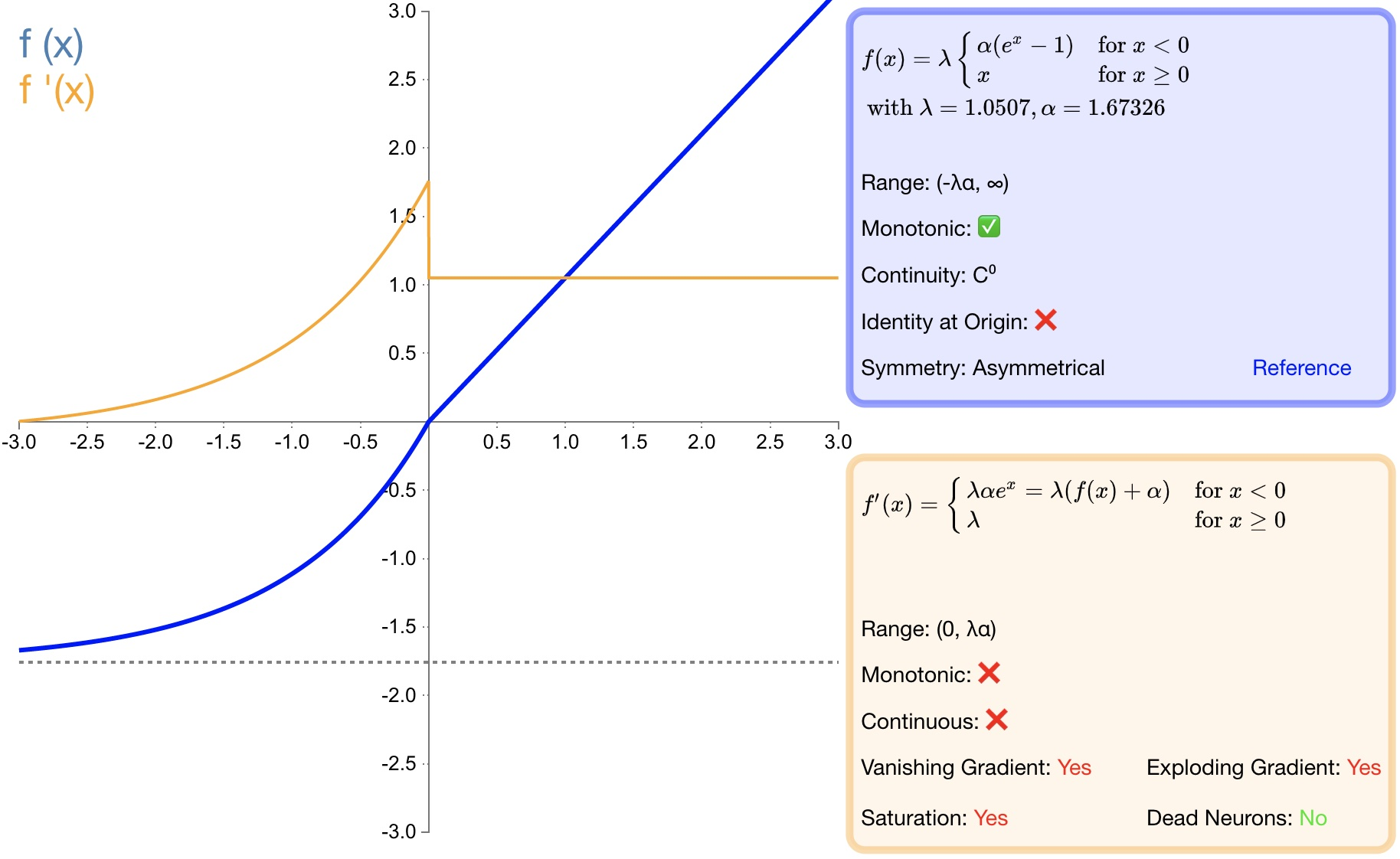1. SELU 是 ELU 的一个变种。其中 λ 和 α 是固定数值（分别为 $$1.0507$$$$1.6726$$）;

2. 经过该激活函数后使得样本分布自动归一化到 $$0$$ 均值和单位方差;

3. 不会出现梯度消失或爆炸问题;

### softsign¶

$f(x)=\frac { x }{ \left| x \right| +1 }$

${ f }^{ ' }(x)=\frac { 1 }{ { (1+\left| x \right| ) }^{ 2 } }$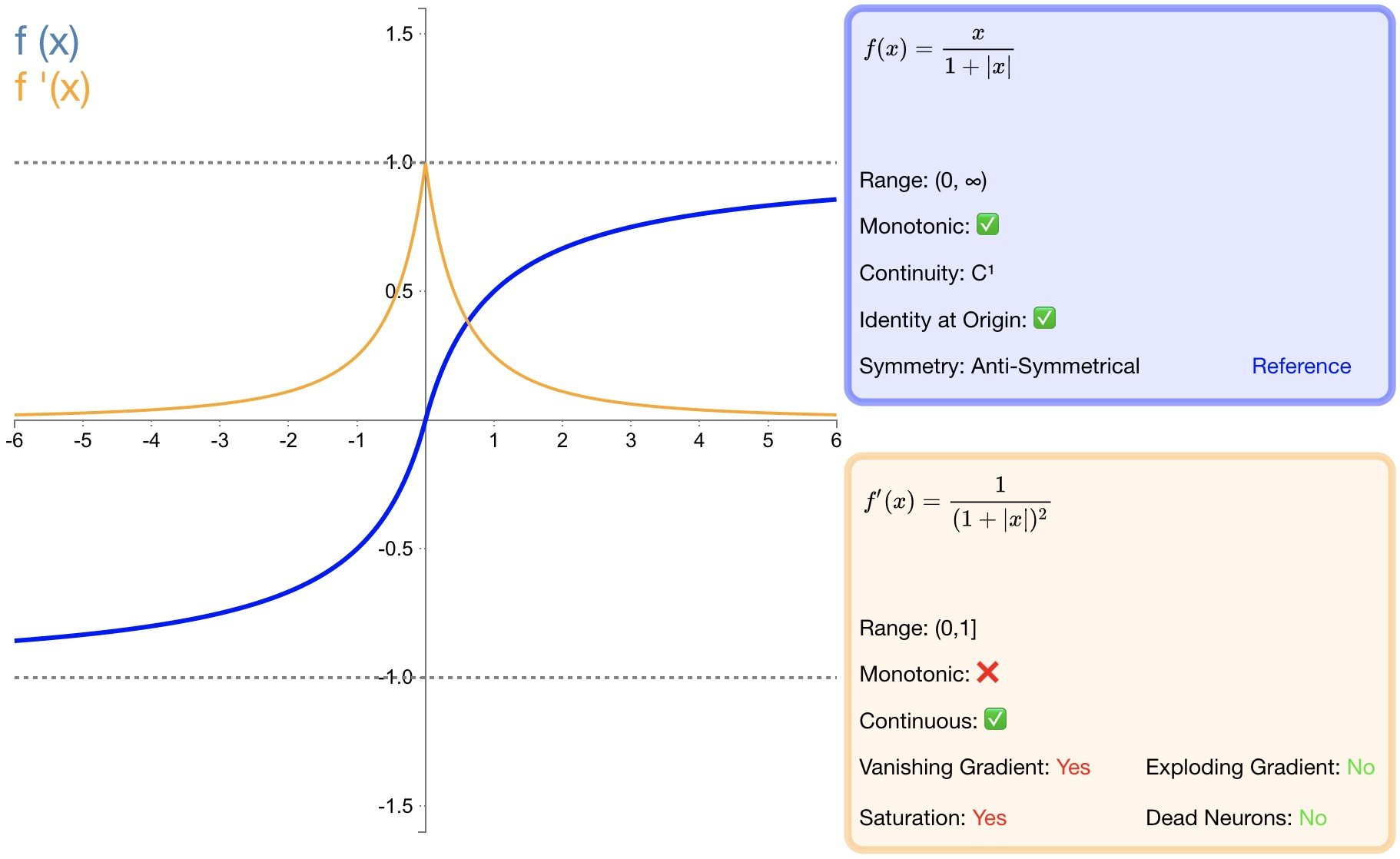1. $$softsign$$$$tanh$$ 激活函数的另一个替代选择；

2. $$softsign$$ 是反对称、去中心、可微分，并返回 $$-1$$$$1$$ 之间的值；

3. $$softsign$$ 更平坦的曲线与更慢的下降导数表明它可以更高效地学习；

1. 导数的计算比$$tanh$$ 更麻烦；

### softplus¶

$f(x)=\ln { (1+{ e }^{ x }) }$

${ f }^{ ' }(x)=\frac { 1 }{ 1+{ e }^{ -x } }$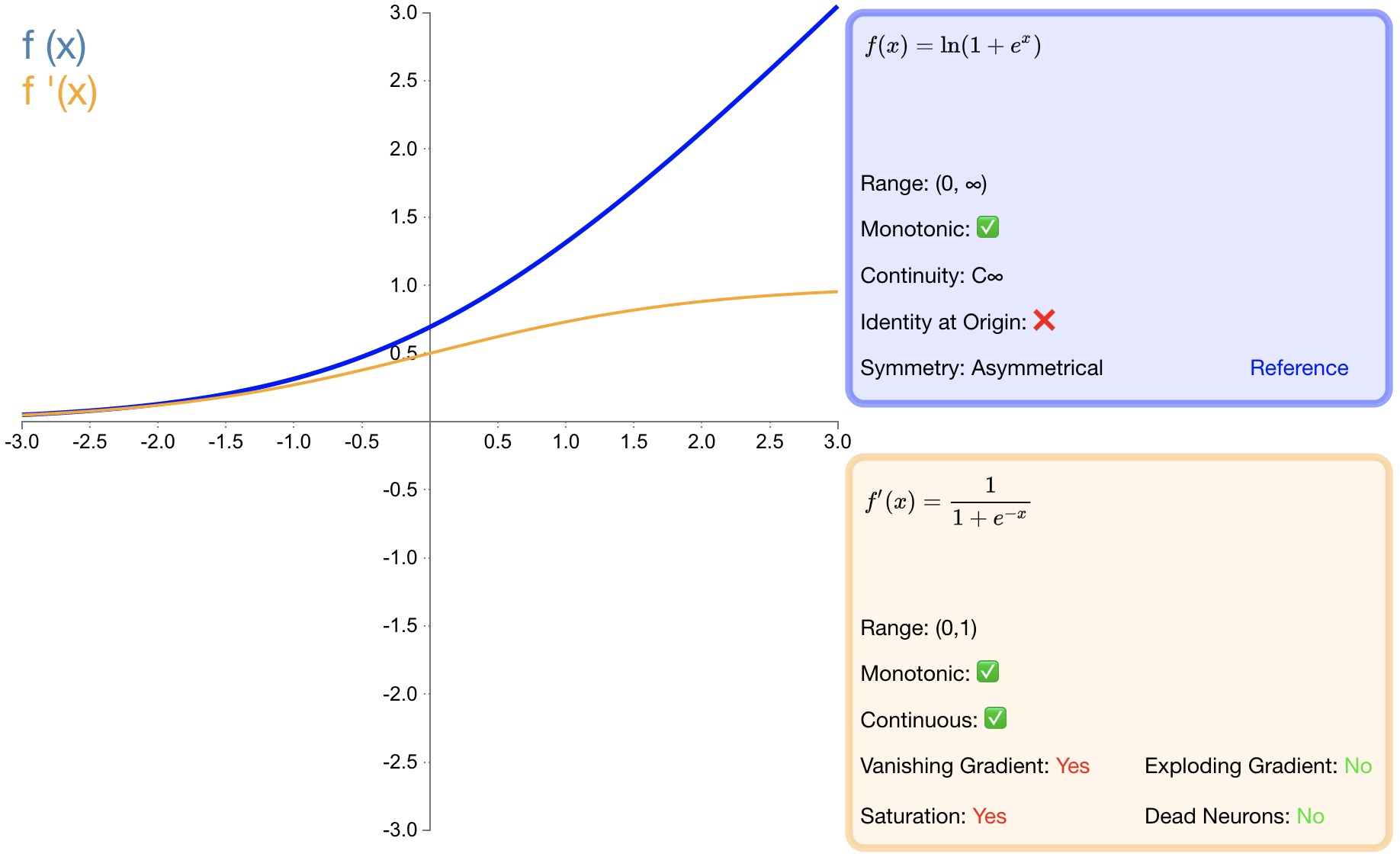1. 作为 $$relu$$ 的一个不错的替代选择，$$softplus$$ 能够返回任何大于 $$0$$ 的值。

2. $$relu$$ 不同，$$softplus$$ 的导数是连续的、非零的，无处不在，从而防止出现死神经元。

1. 导数常常小于 $$1$$ ，也可能出现梯度消失的问题。

2. $$softplus$$ 另一个不同于 $$relu$$ 的地方在于其不对称性，不以零为中心，可能会妨碍学习。

### softmax¶

softmax 函数一般用于多分类问题中，它是对逻辑斯蒂（logistic）回归的一种推广，也被称为多项逻辑斯蒂回归模型(multi-nominal logistic mode)。假设要实现 k 个类别的分类任务，Softmax 函数将输入数据 $$x_i$$ 映射到第 $$i$$ 个类别的概率 $$y_i$$ 如下计算：

$y_i=soft\max \left( x_i \right) =\frac{e^{x_i}}{\sum_{j=1}^k{e^{x_j}}}$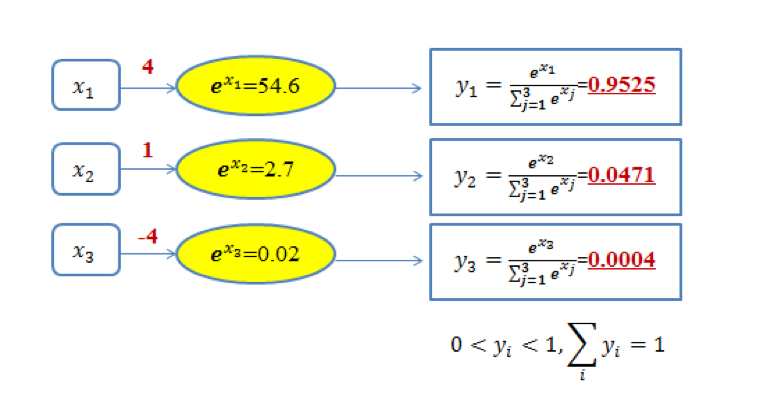### swish¶

$f\left( x \right) =x\cdot \sigma \left( x \right)$

$$swish$$ 激活函数的图形如 图14 所示：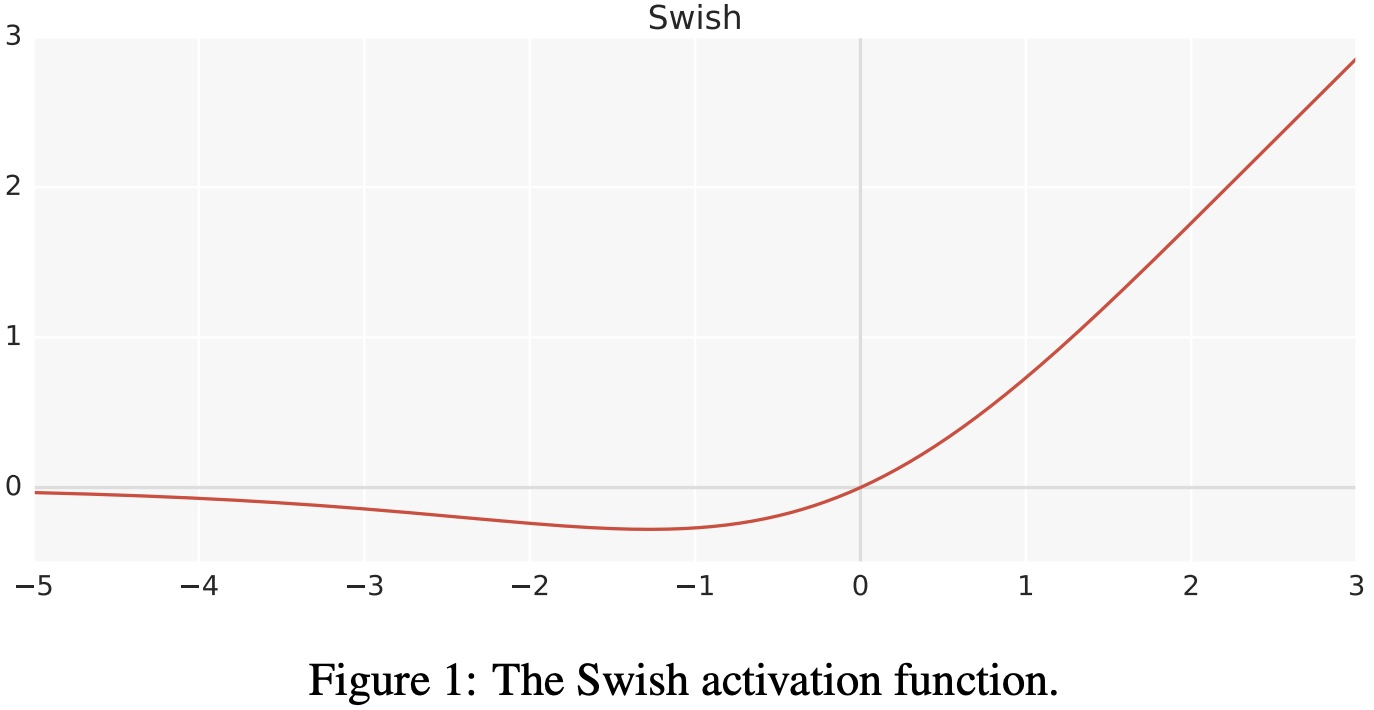$$swish$$ 激活函数的一阶导数如下：

$\begin{split}\begin{array}{c} f^{'}\left( x \right) =\sigma \left( x \right) +x\cdot \sigma \left( x \right) \left( 1-\sigma \left( x \right) \right)\\ =\sigma \left( x \right) +x\cdot \sigma \left( x \right) -x\cdot \sigma \left( x \right) ^2\\ =x\cdot \sigma \left( x \right) +\sigma \left( x \right) \left( 1-x\cdot \sigma \left( x \right) \right)\\ =f\left( x \right) +\sigma \left( x \right) \left( 1-f\left( x \right) \right)\\ \end{array}\end{split}$

$$swish$$ 激活函数的一阶和二阶导数的图形如 图15 所示：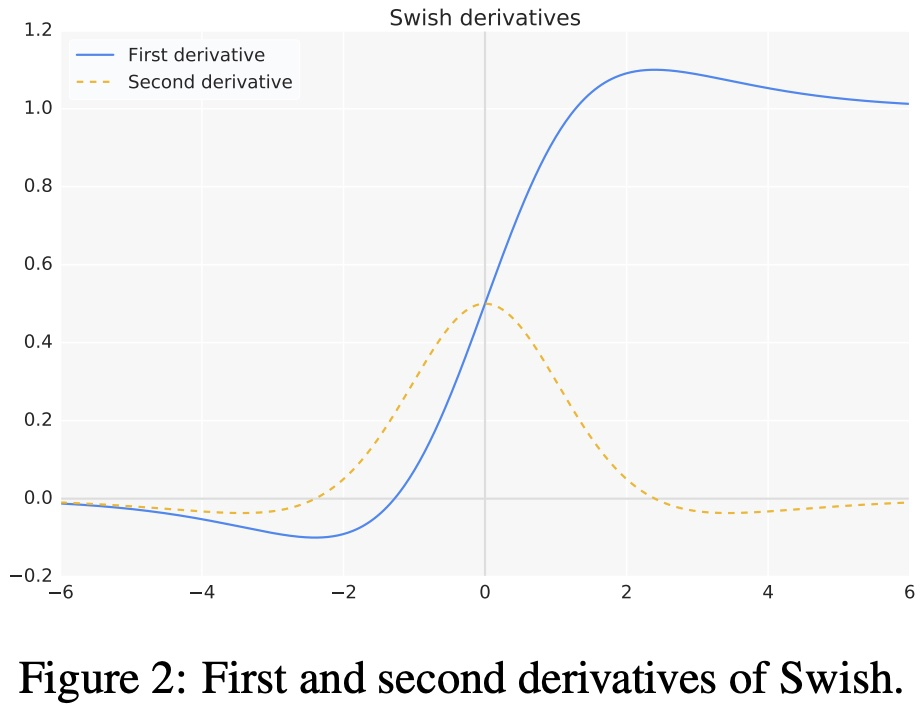$f\left( x \right) =x\cdot \sigma \left( \beta x \right)$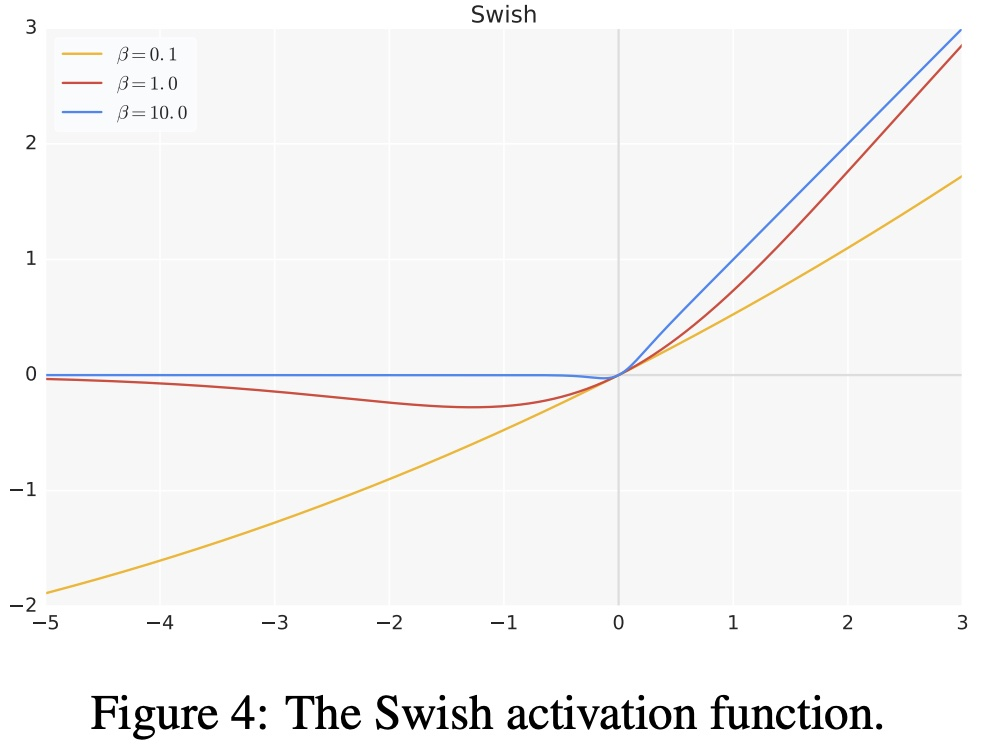1. $$x>0$$ 时，不存在梯度消失的情况；当 $$x<0$$ 时，神经元也不会像 ReLU 一样出现死亡的情况；

2. $$swish$$ 处处可导，连续光滑；

3. $$swish$$ 并非一个单调的函数；

4. 提升了模型的性能；

1. 计算量大；

### hswish¶

$f\left( x \right) =x\frac{\text{Re}LU6\left( x+3 \right)}{6}$

$$hard \ swish$$$$swish$$ 激活函数对比如 图17 所示：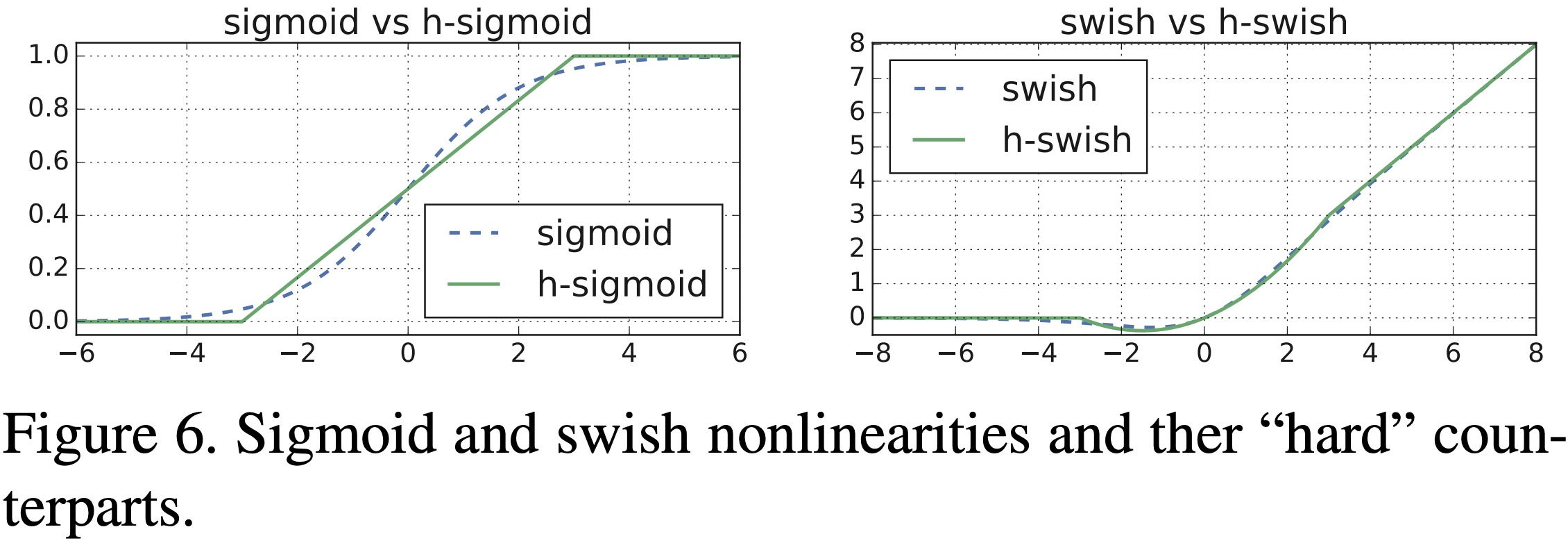## 激活函数的选择¶

1. 浅层网络在分类器时，$$sigmoid$$ 函数及其组合通常效果更好。

2. 由于梯度消失问题，有时要避免使用 $$sigmoid$$$$tanh$$ 函数。

3. $$relu$$ 函数是一个通用的激活函数，目前在大多数情况下使用。

4. 如果神经网络中出现死神经元，那么 $$prelu$$ 函数就是最好的选择。

5. $$relu$$ 函数只能在隐藏层中使用。

6. 通常，可以从 $$relu$$ 函数开始，如果 $$relu$$ 函数没有提供最优结果，再尝试其他激活函数。

## 激活函数相关问题¶

$\tan\text{h}^{'}\left( x \right) =1-\tan\text{h}\left( x \right) ^2\in \left( 0,1 \right)$
$s^{'}\left( x \right) =s\left( x \right) \left( 1-s\left( x \right) \right) \in \left( 0,\frac{1}{4} \right]$

sigmoid 和 softmax 有什么区别？

1. 二分类问题时 $$sigmoid$$$$softmax$$ 是一样的，都是求 $$cross \ entropy \ loss$$ ，而 $$softmax$$ 可以用于多分类问题。

2. $$softmax$$$$sigmoid$$ 的扩展，因为，当类别数 $$k=2$$ 时，$$softmax$$ 回归退化为 $$logistic$$ 回归。

3. $$softmax$$ 建模使用的分布是多项式分布，而 $$logistic$$ 则基于伯努利分布。

4. 多个 $$logistic$$ 回归通过叠加也同样可以实现多分类的效果，但是 $$softmax$$ 回归进行的多分类，类与类之间是互斥的，即一个输入只能被归为一类；多 $$logistic$$ 回归进行多分类，输出的类别并不是互斥的，即”苹果”这个词语既属于”水果”类也属于”$$3C$$”类别。